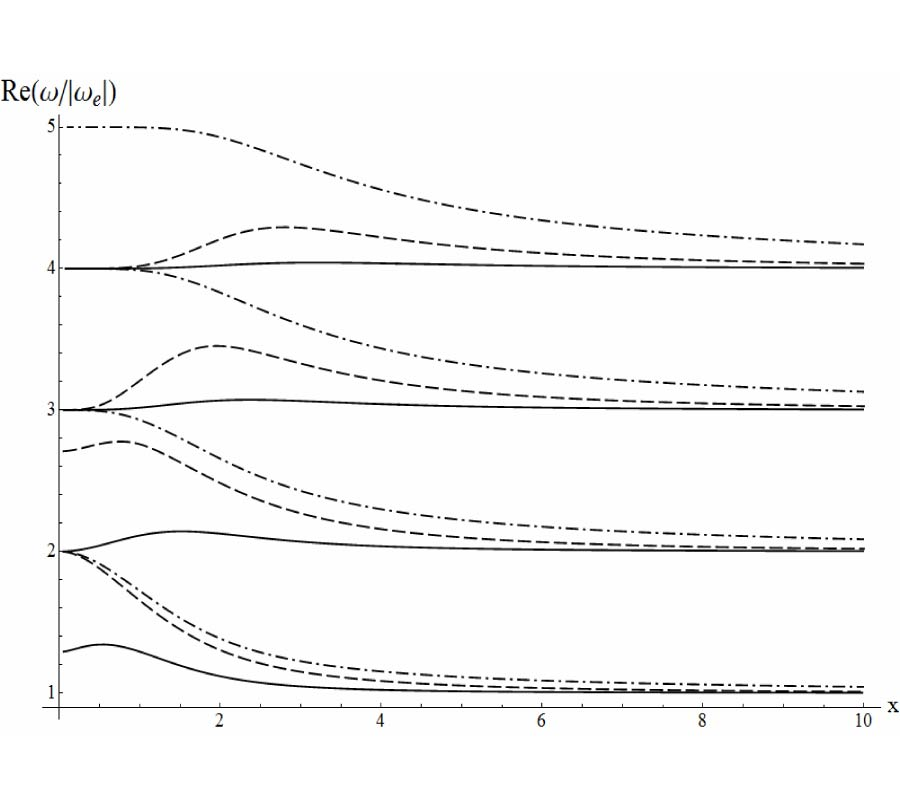Vol. 34
Latest Volume
All Volumes
All Journals
2013-11-25
Parametric Instability of Surface Electron Cyclotron TM-Modes
By
Progress In Electromagnetics Research M, Vol. 34, 39-46, 2014
Abstract
Excitation of waves at harmonics of electron cyclotron frequency due to utilization of an external alternating electric field is under the consideration. It is proved that they are eigen modes of plasma-dielectric-metal structures in both long (as compared with electron Larmor radius) wavelength range and short wavelength range if an external steady magnetic field is oriented perpendicularly to the plasma interface. It is assumed that uniform external electric field operates at the frequency, which belongs to the range of electron cyclotron frequencies. The problem is solved theoretically using kinetic Vlasov-Boltzmann equation for description of the plasma particles motion and Maxwell equations for description of TM polarized field of these modes. Non-linear boundary condition for tangential magnetic field of these TM-modes is formulated using conception of non-linear surface electric current. Infinite set of equations for harmonics of their tangential electric field is derived due to this condition. This set is solved using approach of the wave packet consisting of the basic harmonic and two nearest satellite harmonics. Simple analytical expression for growth rate of surface electron cyclotron TM-modes' parametric instability is obtained and analyzed numerically.
Citation
Volodymyr Girka, and Vitalii Viktorovych Iarko, "Parametric Instability of Surface Electron Cyclotron TM-Modes," Progress In Electromagnetics Research M, Vol. 34, 39-46, 2014.
doi:10.2528/PIERM13091702
References

1. Akhiezer, A. I., I. A. Akhiezer, R. V. Polovin, A. G. Sitenko, and K. N. Stepanov, Plasma Electrodynamics, Pergamon Press, Oxford, 1975.

Download Full Article (158) View Full Article